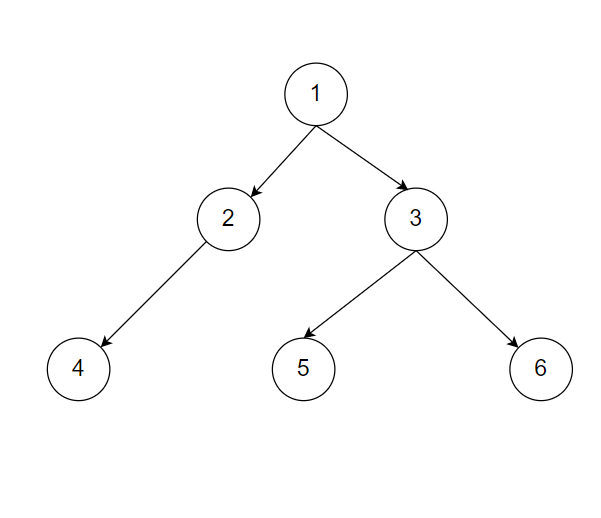New update is available. Click here to update.

# Find The Sum Of The Left Leaves

Last Updated: 29 Mar, 2021
Difficulty: Moderate

## PROBLEM STATEMENT

#### In a binary tree, a leaf is a node such that it does not have any children. Node ‘1’ is always the root of the binary tree. Left leaves are those nodes that are the left child of their parent and a leaf node.

##### Example:
``````Let’s say you have a binary tree as follows:-
``````#### Node 4 and Node 5 are leaf nodes and left child of their parent. Node 6 is a leaf node but not the left child of its parent node 3. Therefore return ‘4+5= 9’ as the answer.

##### Note:
``````You do not need to print anything; it has already been taken care of. Just implement the function.
``````
##### Input Format:
``````The first line of input contains an integer ‘t’ denoting the number of test cases.
The next ‘t’ lines represent the ‘t’ test cases.

The first line of input contains the elements of the tree in the level order form separated by a single space.
If any node does not have a left or right child, take -1 in its place. Refer to the example below.

Example:

Elements are in the level order form. The input consists of values of nodes separated by a single space in a single line. In case a node is null, we take -1 in its place.

For example, the input for the tree depicted in the below image would be :
```````````` 1
2 3
4 -1 5 6
-1 7 -1 -1 -1 -1
-1 -1
``````
##### Explanation :
``````Level 1 :
The root node of the tree is 1

Level 2 :
Left child of 1 = 2
Right child of 1 = 3

Level 3 :
Left child of 2 = 4
Right child of 2 = null (-1)
Left child of 3 = 5
Right child of 3 = 6

Level 4 :
Left child of 4 = null (-1)
Right child of 4 = 7
Left child of 5 = null (-1)
Right child of 5 = null (-1)
Left child of 6 = null (-1)
Right child of 6 = null (-1)

Level 5 :
Left child of 7 = null (-1)
Right child of 7 = null (-1)

The first not-null node (of the previous level) is treated as the parent of the first two nodes of the current level. The second not-null node (of the previous level) is treated as the parent node for the next two nodes of the current level and so on.

The input ends when all nodes at the last level are null (-1).
``````
##### Note :
``````The above format was just to provide clarity on how the input is formed for a given tree.

The sequence will be put together in a single line separated by a single space. Hence, for the above-depicted tree, the input will be given as:

1 2 3 4 -1 5 6 -1 7 -1 -1 -1 -1 -1 -1
``````
##### Output Format:
``````For each test case return the sum of left leaves.
``````
##### Constraints:
``````1 <= T <= 10
1 <= N <= 1000

Where ‘T’ is the number of test cases.‘N’ is the number of nodes in the binary tree.

Time Limit: 1sec
``````## Approach 1

We will start from the root and check if the node has the left child, if yes then we will check if the node is a leaf node, if it is a leaf node then we will add it to the answer. We will push all the children of the current node in the queue and iteratively visit all the children.

We will apply the algorithm as follows:-

• Declare a queue ‘q’ which stores nodes whose children are not yet visited.
• Declare ‘ans’ and initialize it with ‘0’.
• Insert node ‘1’ i.e root in the queue ‘q’.
• While queue is not empty:-
• Store the front of the queue in ‘u’
• Pop the front of the queue ‘q’.
• If ‘u’ has the left child, check if the left child of ‘u’ has any child. If the left child of ‘u’ does not have any child add it to ‘ans’ as it is a left leaf node.
• Insert all the children of ‘u’ into the queue ‘q’.
• Return ‘ans’.